## Example Questions

### Example Question #102 : Coordinate Geometry

Which of the following terms are linear?

x2

all of these terms are linear

x

yz

sin(x)

x

Explanation:

Linear terms have only one variable in a product and no exponents other than 0 or 1. x2 has an exponent other than 0 or 1 so it is not linear. yz has two variables so is also not a linear term. Linear terms cannot have functions of variables either, so sin(x) is not linear.

We can also think of these terms somewhat like graphing equations. Linear equations are straight lines. You might recognize, for example, that x2 should be a parabola. Sin(x) has a graph that looks like a harmonic wave. Clearly these two shaps aren't straight lines!

### Example Question #102 : Coordinate Geometry

The slope of a line segment with points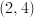and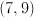is: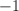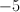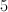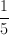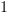Explanation:

The formula for calculating slope is rise over run, or the difference in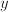divided by the difference in. In this case, the difference inis 5 while the difference inis 5, resulting in a slope of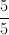or 1.

### Example Question #3 : Graphing

What is the slope of the linear line that passes through the origin and the point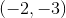?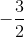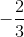Explanation:

Slope of a line given 2 points can be found using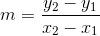.

Therefore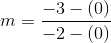or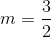Tired of practice problems?

Try live online GRE prep today.